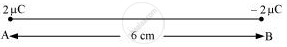# Two Charges 2 μC and −2 µC Are Placed at Points a and B 6 cm Apart. (A) Identify an Equipotential Surface of the System. - Physics

Numerical

Two charges 2 μC and −2 µC are placed at points A and B 6 cm apart.

(a) Identify an equipotential surface of the system.

(b) What is the direction of the electric field at every point on this surface?

#### Solution

(a) The situation is represented in the given figure.An equipotential surface is a plane on which total potential is zero everywhere. This plane is normal to line AB. The plane is located at the mid-point of line AB because the magnitude of charges is the same.

(b) The direction of the electric field at every point on this surface is normal to the plane in the direction of AB.

Concept: Equipotential Surfaces
Is there an error in this question or solution?
Chapter 2: Electrostatic Potential and Capacitance - Exercise [Page 86]

#### APPEARS IN

NCERT Physics Part 1 and 2 Class 12
Chapter 2 Electrostatic Potential and Capacitance
Exercise | Q 2.3 | Page 86
NCERT Class 12 Physics Textbook
Chapter 2 Electrostatic Potential and Capacitance
Exercise | Q 3 | Page 87
Share